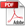# Application Developer's Guide — Chapter 20

## Machine Learning with the CNTK API

This chapter contains the following sections:

### Overview of Machine Learning

Machine learning can be conveniently perceived as a function approximator. There is an indescribable law that determines if a picture is a picture of a cat, or if the price of a stock will go up tomorrow, and machine learning can approximate that law (with various degrees of accuracy). The law itself is a blackbox that takes input and produces output. For image classification, the input is pixel values and the output is cat or not; for a stock price, the input is stock trades and the output is price. A machine learning model takes input in a form understandable by the machine (high dimensional matrix of numbers, called tensors), performs a series of computation on the input, and then produces an output. The machine learns from comparing its output to the ground truth (the output of that law), and adjust its computations of the input, to produce better output that is closer to the ground truth.

Consider again the example of image classification. A simple machine learning model can be like this: convert the image into a matrix of pixel values `x`; multiply it with another matrix `W`. If the result `Wx` is larger than a Threshold, it's a cat, otherwise it's not. For the model to succeed, it needs labeled training data of images. The model starts with a totally random matrix `W`, and produces output on all training images. It will make lots of mistakes, and for every mistake it makes, it adjusts `W` so that the output `Wx` is closer to the ground truth label. The precise amount of adjustment of `W` is determined through a process called error back propagation. In the example described here, the computation is a simple one matrix multiplication; however, in real world applications, you can have hundreds of layers of computations, with millions of different `W` parameters.

The MarkLogic approach to machine learning is to accelerate and improve the data curation life cycle by developing models using high quality data. Bad inputs result in bad outputs (garbage in = garbage out). In the case of machine learning, the model used to convert input to output is written by the machine itself during training, and that is based on the training input. Bad training data can damage the model in ways you cannot understand, rendering it useless. Because the models are opaque, you may not even know they are damaged. You don't use machine learning to solve easy problems and hard questions answered wrong are hard to identify. MarkLogic has many features, such as the Data Hub Framework and Entity Services, you can leverage to ensure the quality of the data used to create your models.

The MarkLogic Machine Learning CNTK API implements the Microsoft Cognitive Toolkit (CNTK) framework described in https://docs.microsoft.com/en-us/cognitive-toolkit/. Computing the output based on input `x` is called a forward pass and it is the evaluation part of CNTK. Adjusting the network parameters `W` based on error back propagation is called a backward pass, which is where training happens.

CNTK is at its core a high performance tensor (high dimensional matrix) library. The core functionality is:

• The composition of computation network
• (Training) Auto differentiation for error back propagation
• Highly optimized kernels to perform said computation on different devices.

### Terms

The material in this guide assumes you are familiar with the basic concepts of machine learning. Some terms have ambiguous popular definitions, so they are described below.

Term Definition
Artificial Intelligence Any technique which enables computers to mimic human behavior
Machine Learning Subset of AI techniques which use mathematical methods (commonly statistics or liner algebra) to modify behavior with execution.
Deep Learning

Subset of Machine Learning which makes the computation of neural networks feasible.

Deep Learning is associated with a machine learning algorithm (Artificial Neural Network, ANN) which uses the concept of human brain to facilitate the modeling of arbitrary functions. ANN requires a vast amount of data and this algorithm is highly flexible when it comes to model multiple outputs simultaneously. To understand ANN in detail, see https://www.analyticsvidhya.com/blog/2014/10/ann-work-simplified/.

Accuracy

Accuracy is a metric by which one can examine how good is the machine learning model. Let us look at the confusion matrix to understand it in a better way: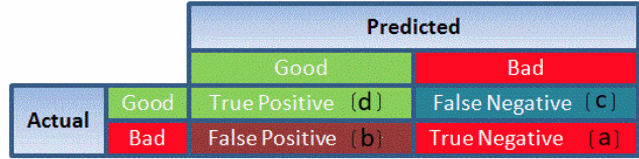So, the accuracy is the ratio of correctly predicted classes to the total classes predicted. Here, the accuracy will be:

```               True Positive + True Negatives
__________________________________________________________________```
`True Positive + True Negatives + False Positives + False Negatives`
Autoregression

Autoregression is a time series model that uses observations from previous time steps as input to a regression equation to predict the value at the next time step. The autoregressive model specifies that the output variable depends linearly on its own previous values. In this technique input variables are taken as observations at previous time steps, called lag variables.

For example, we can predict the value for the next time step (t+1) given the observations at the last two time steps (t-1 and t-2). As a regression model, this would look as follows:

`X(t+1) = b0 + b1*X(t-1) + b2*X(t-2)`

Since the regression model uses data from the same input variable at previous time steps, it is referred to as an autoregression.

Back Propagation In neural networks, if the estimated output is far away from the actual output (high error), we update the biases and weights based on the error. This weight and bias updating process is known as Back Propagation. Back-propagation (BP) algorithms work by determining the loss (or error) at the output and then propagating it back into the network. The weights are updated to minimize the error resulting from each neuron. The first step in minimizing the error is to determine the gradient (Derivatives) of each node w.r.t. the final output.
Bayes' Theorem

Bayes' theorem is used to calculate the conditional probability. Conditional probability is the probability of an event 'B' occurring given the related event 'A' has already occurred.

For example, a clinic wants to cure cancer of the patients visiting the clinic.

• A = an event Person has cancer
• B = an event Person is a smoker

The clinic wishes to calculate the proportion of smokers from the ones diagnosed with cancer.

Use the Bayes' Theorem (also known as Bayes' rule) as follows: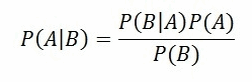To understand Bayes' Theorem in detail, refer to http://faculty.washington.edu/tamre/BayesTheorem.pdf.

Classification Threshold Classification threshold is the value which is used to classify a new observation as 1 or 0. When we get an output as probabilities and have to classify them into classes, we decide some threshold value and if the probability is above that threshold value we classify it as 1, and 0 otherwise. To find the optimal threshold value, one can plot the AUC-ROC and keep changing the threshold value. The value which will give the maximum AUC will be the optimal threshold value.
Clustering

Clustering is an unsupervised learning method used to discover the inherent groupings in the data. For example: Grouping customers on the basis of their purchasing behavior which is further used to segment the customers. And then the companies can use the appropriate marketing tactics to generate more profits.

Example of clustering algorithms: K-Means, hierarchical clustering, etc.

Confidence Interval A confidence interval is used to estimate what percent of a population fits a category based on the results from a sample population. For example, if 70 adults own a cell phone in a random sample of 100 adults, we can be fairly confident that the true percentage amongst the population is somewhere between 61% and 79%. For more information, see https://www.analyticsvidhya.com/blog/2015/09/hypothesis-testing-explained/.
Convergence Convergence refers to moving towards union or uniformity. An iterative algorithm is said to converge when as the iterations proceed the output gets closer and closer to a specific value.
Correlation

Correlation is the ratio of covariance of two variables to a product of variance (of the variables). It takes a value between +1 and -1. An extreme value on both the side means they are strongly correlated with each other. A value of zero indicates a NIL correlation but not a non-dependence. You'll understand this clearly in one of the following answers.

The most widely used correlation coefficient is Pearson Coefficient. Here is the mathematical formula to derive Pearson Coefficient.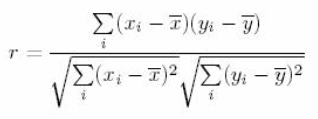Decision Boundary

In a statistical-classification problem with two or more classes, a decision boundary or decision surface is a hyper surface that partitions the underlying vector space into two or more sets, one for each class. How well the classifier works depends upon how closely the input patterns to be classified resemble the decision boundary. In the example sketched below, the correspondence is very close, and one can anticipate excellent performance.

Here the lines separating each class are decision boundaries.Dimensionality Reduction Dimensionality Reduction is the process of reducing the number of random variables under consideration by obtaining a set of principal variables. Dimension Reduction refers to the process of converting a set of data having vast dimensions into data with lesser dimensions ensuring that it conveys similar information concisely. Some of the benefits of dimensionality reduction:
• It helps in data compressing and reducing the storage space required
• It fastens the time required for performing same computations
• It takes care of multicollinearity that improves the model performance. It removes redundant features
• Reducing the dimensions of data to 2D or 3D may allow us to plot and visualize it precisely
• It is helpful in noise removal also and as result of that we can improve the performance of models
Datasets

Training data is used to train a model. It means that ML model sees that data and learns to detect patterns or determine which features are most important during prediction.

Validation data is used for tuning model parameters and comparing different models in order to determine the best ones. The validation data must be different from the training data, and must not be used in the training phase. Otherwise, the model would overfit, and poorly generalize to the new (production) data.

Test data is used once the final model is chosen to simulate the model's behavior on a completely unseen data, i.e. data points that weren't used in building models or even in deciding which model to choose.

Ground Truth The reality you want your model to predict.
Model A machine-created object that takes input in a form understandable by the machine, performs a series of computation on the input, and then produces an output. The model is built from repeatedly comparing its output to the ground truth and adjusting its computations of the input to produce better output that is closer to the ground truth.
Unrulier Network

Neural Networks is a very wide family of Machine Learning models. The main idea behind them is to mimic the behavior of a human brain when processing data. Just like the networks connecting real neurons in the human brain, artificial neural networks are composed of layers. Each layer is a set of neurons, all of which are responsible for detecting different things. A neural network processes data sequentially, which means that only the first layer is directly connected to the input. All subsequent layers detect features based on the output of a previous layer, which enables the model to learn more and more complex patterns in data as the number of layers increases. When a number of layers increases rapidly, the model is often called a Deep Learning model. It is difficult to determine a specific number of layers above which a network is considered deep, 10 years ago it used to be 3 and now is around 20.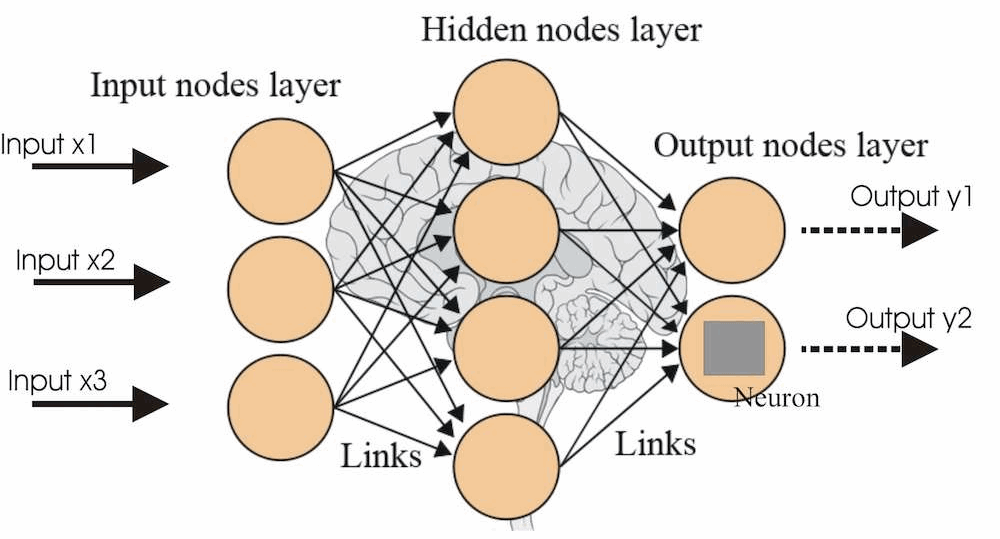There are many types of Neural Networks. A list of the most common can be found https://en.wikipedia.org/wiki/Types_of_artificial_neural_networks.

Threshold

A threshold is a numeric value used to determine whether the computed output is a match.

Most of the time the value of a threshold is obtained through training. The initial value can be chosen randomly, for example 2.2, then the training algorithm finds out that most of the predictions are wrong (cats classified as dogs), then the training algorithm adjusts the value of the threshold, so that the prediction can be more accurate.

Sometimes the threshold is determined manually, like in our current smart mastering implementation. They have a combined score, describing similarity between two entities. If the score is larger than a threshold, the two entities can be considered a match. That threshold is pre-determined, manually. No training is involved.

### Types of Machine Learning

This section describes the types of machine learning:

#### Supervised learning

Supervised learning is a family of Machine Learning models that teach themselves by example. This means that data for a supervised ML task needs to be labeled (assigned the right, ground-truth class). For instance, if we would like to build a Machine Learning model for recognizing if a given text is about marketing, we need to provide the model with a set of labeled examples (text + information if it is about marketing or not). Given a new, unseen example, the model predicts its target ...Äì e.g. for the stated example, a label (e.g., 1 if a text is about marketing and 0 otherwise).

#### Unsupervised Learning

Contrary to Supervised Learning, Unsupervised Learning models teach themselves by observation. The data provided to that kind of algorithms is unlabeled (there is no ground truth value given to the algorithm). Unsupervised learning models are able to find the structure or relationships between different inputs. The most important kind of unsupervised learning techniques is clustering. In clustering, given the data, the model creates different clusters of inputs (where similar inputs are in the same clusters) and is able to put any new, previously unseen input in the appropriate cluster.

#### Reinforcement Learning

Reinforcement Learning (RL) differs in its approach from the approaches we've described earlier. In RL the algorithm plays a game, in which it aims to maximize the reward. The algorithm tries different approaches moves using trial-and-error and sees which one boost the most profit. The most commonly known use cases of RL are teaching a computer to solve a Rubik's Cube or play chess, but there is more to Reinforcement Learning than just games. Recently, there is an increasing number of RL solutions in Real Time Bidding, where the model is responsible for bidding a spot for an ad and its reward is the client's conversion rate.

### Example CNTK Applications

This section describes two example CNTK applications:

#### Example Application using an Existing Model

Download the Sample text summarization model and Vocabulary file for text summarizer from the Machine Learning Models section on https://developer.marklogic.com/products.

Using the Query Console, select `Documents` as the Database and `XQuery` as the Query Type and run the following query to load the `sample_text_summarizer.model` file into the `modules` database, and the `sample_text_summarizer_vocab.txt` file into the `Documents` database:

```xquery version "1.0-ml";

"/home/mldev/Models/`sample_text_summarizer.model`",
map:map() => map:with("uri", "/Models/greedy_model.model")
=> map:with("format", "binary"))'
map:map() => map:with("database", xdmp:modules-database()))
,
"/home/mldev/Models/`sample_text_summarizer_vocab`.txt",
<uri>/Models/vocab_comma.txt</uri>
</options> ))```

The `sample_text_summarizer.model` file is the pre-built model file. The `sample_text_summarizer_vocab.txt` file contains all of the possible tokens (meaningful combinations of letters/special characters like #,.: and so on) of the English language.

The following example uses the model to summarize the text assigned to the `\$article` variable. In order for the input article to be understood by the model, the code uses the `sample_text_summarizer.txt` file to transform the English article into a numeric matrix/vector. Each token in the article is replaced by its position in the vocabulary file. For example, if the input article is Dog is cute and the vocabulary file consists of dog, cat, snake, is, are, cute, evil, the input article is vectorized to [1,4,6].

The cntk:function constructs the model from the `sample_text_summarizer.model` file and stores it as an axis object. The cntk:function-arguments and cntk:function-output functions extract the input and output variables from the model, respectively. The fn:tokenize and `cntk:variable-shape` functions vectorizes and shapes the input. The cntk:sequence function constructs a sequence of values to be used as input to the model.

The cntk:evaluate function evaluates the input against the model and returns a sequence of cntk:value, in the same order as the sequence of output variables.

```xquery version "1.0-ml";

(: Input text to be summarized :)
let \$article :=
"<s> a moderate earthquake jolted southern iran on wednesday,
killing six people, and damaging scores of homes
on a resort island in strategic gulf waters. </s>"

(: Load a pretrained model from the database, and add tweaks :)
let \$model_doc := xdmp:eval('fn:doc("/Models/greedy_model.model")',(),
map:map() => map:with("database", xdmp:modules-database()))
let \$model := cntk:argmax(
cntk:function(\$model_doc/binary()),
cntk:axis(0))

(: Extract required variables from the model :)
let \$input-variable := cntk:function-arguments(\$model)
let \$output-variable := cntk:function-output(\$model)

(: Vectorize the input text, preparing input values for the model :)
let \$vocab := fn:tokenize(
fn:doc("/Models/vocab_comma.txt")/text(),",")
let \$tokens := fn:tokenize(\$article, " ")
let \$sequences :=
for \$token in \$tokens return fn:index-of(\$vocab, \$token)+1
let \$one-hot-sequences :=
for \$idx in \$sequences
return ((for \$_ in (1 to \$idx - 1) return 0), 1,
(for \$_ in (\$idx+1 to 122920) return 0))
let \$shape := cntk:variable-shape(\$input-variable)
let \$input-value := cntk:sequence(
\$shape,
json:to-array(\$one-hot-sequences),
fn:true())
let \$input-pair := json:to-array((\$input-variable, \$input-value))

(: Perform evaluation of the model on our input text :)
let \$output-value := cntk:evaluate(
\$model,
\$input-pair,
(\$output-variable))
let \$output-sequence := json:array-values(
cntk:value-to-array(\$output-variable,
\$output-value),
fn:true())

(: Print out the output of the model, which is a summarization of our input text :)
return fn:string-join(
for \$output in \$output-sequence return \$vocab[\$output], " ")```

The output will look like the following:

`moderate earthquake rocks iran </s>`

#### Example Application that Creates a Model

The example below first constructs a very simple model and then trains the model. The model is simply a multiplication of a cntk:parameter (`\$W`) and the input variable (`\$input-variable`). The output is compared to the ground truth, and the model parameter (`\$W`) is updated by the trainer. The cntk:train-minibatch function is executed ten times to do the training. The returned values are the loss value, which describes how far the predictions are from the ground truth labels. The smaller the loss value, the better the model is performing.

```xquery version "1.0-ml";

(: Set up environment, parameters :)
let \$input-dims := 2
let \$num-classes := 2
let \$input-variable :=
cntk:input-variable(cntk:shape((\$input-dims)),
"float")
let \$training-data :=
json:to-array((
2.2741797,
3.56347561,
5.12873602,
5.79089499,
1.3574543,
5.5718112,
3.54340553,
2.46254587))
let \$input-value := cntk:batch(
cntk:shape((\$input-dims)),
\$training-data)
let \$labels-variable :=
cntk:input-variable(cntk:shape((\$input-dims)),
"float")
let \$labels := json:to-array((1,0,0,1,0,1,1,0))
let \$labels-value := cntk:batch(cntk:shape((\$num-classes)), \$labels)

(: Construct the model :)
let \$W := cntk:parameter(
cntk:shape((\$input-dims)),
"float",
cntk:glorot-uniform-initializer())
let \$model := cntk:times(\$input-variable, \$W, 1, -1)

(: Set up training parameters, and do the training :)
let \$learner :=
cntk:sgd-learner((\$W),
cntk:learning-rate-schedule-from-constant(0.1))
let \$loss := cntk:cross-entropy-with-softmax(
\$model,
\$labels-variable,
cntk:axis(-1))
let \$trainer := cntk:trainer(\$model, (\$learner), \$loss)
let \$input-pair := json:to-array((\$input-variable, \$input-value))
let \$labels-pair := json:to-array((\$labels-variable, \$labels-value))
let \$minibatch := json:to-array((\$input-pair, \$labels-pair))
let \$loss-values :=
for \$i in (1 to 10)
let \$__ := cntk:train-minibatch(\$trainer, \$minibatch, fn:false())
return cntk:previous-minibatch-loss-average(\$trainer)
return \$loss-values```

The output will look like the following:

```1.98877370357513
0.763397812843323
0.695295095443726
0.627196073532105
0.559098362922668
0.491001456975937
0.422904640436173
0.354807853698731
0.286710739135742
0.218613743782043```

#### Security Considerations

The CNTK subsystem is not allowed to read or write the database or the filesystem. A small number of built-in functions (like cntk:evaluate and cntk:session-run) are allowed to run a program, and for that, they will require a set of special privileges.

A CNTK model is basically an arbitrary program, so take appropriate care before executing these programs. Built-in functions that read and write the database will require special privileges.

The following functions will require special privileges.

CNTK Functions & Privileges
Function Name Privilege Name Privilege Action Privilege Type
cntk:evaluate cntk:evaluate http://marklogic.com/cntk/privileges/cntk-evaluate execute
cntk:train-minibatch cntk:train-minibatch http://marklogic.com/cntk/privileges/cntk-train-minibatch execute
cntk:function cntk:function http://marklogic.com/cntk/privileges/cntk-function execute

The above privileges are assigned to the `cntk-user` role. A user must have the `cntk-user` role in order to execute these functions.

#### Configuring the Machine Learning Device

Beginning with MarkLogic version 10.0-2, it is possible to configure the default compute device on which model evaluation takes place. Please see Configuring the Machine Learning Device in the Administrator's Guide for configuration details.

In addition, four built-in functions are added to set and get these configurations, under the admin namespace. They are:

Group Functions for Machine Learning Device
Function Name Functionality

Set the cntk-default-device for the group.

Get the cntk-default-device for the group.

Any change to the configuration caused by using either `admin:group-set-cntk-default-device` or admin:group-set-cntk-gpu-id requires a restart of the MarkLogic server to take effect.